Improve math skills of your kids - Learn step-by-step arithmetic from Math games

Math: Unknown - Step-by-step math calculation game for iOS.

Math: Unknown is much more than a math game. It is a step-by-step math calculation game which will teach users how to calculate in the correct order rather than just asking only the final calculated results.

The app consists of four basic arithmetic operations which are addition, subtraction, multiplication and division. In order to get started, users who are new to arithmetic can learn from animated calculation guides showing step-by-step procedures of solving each type of operation. It is also helpful for experienced users as a quick reference.

Generally, addition and subtraction may be difficult for users who just start learning math especially when questions require carrying or borrowing (also called regrouping). The app helps users to visualize the process of carrying and borrowing in the way it will be done on paper. Once users understand how these operations work, they are ready to learn multiplication and division.

For most students, division is considered as the most difficult arithmetic operation to solve. It is a common area of struggle since it requires prior knowledge of both multiplication and subtraction. To help users understand division, the app uses long division to teach all calculation procedures. Relevant multiplication table will be shown beside the question. Users will have to pick a number from the table which go into the dividend. Multiplication of selected number and divisor is automatically calculated, but the users have to do subtraction and drop down the next digit themselves. Learning whole calculation processes will make them master it in no time.

Math: Unknown is a helpful app for students who seriously want to improve arithmetic calculation skills.

Stresses and Deformations in Beams

Reactions are the forces and/or couples acting at the supports and holding the beam and holding the beam in place. In some cases the user should enter a distributed load to account for the weight of the beam.

The shear V effective on a section is the algebraic sum of all forces acting parallel to and on one side of the section,The bending moment is the algebraic sum of the moments due to applied loads and other applied moments to one side of the section of interest. Using value V bending moment can be calculated
where
x = position on the beam measured along its length
M0 = constant of integration evaluated from the boundary conditions.
A bending moment that bends a beam convex downward (tensile stress on bottom fiber) is considered positive, while convex upward (compressive on bottom fiber) is negative.Moment and shear diagram constructed by plotting to scale the particular entity as the ordinate for each section of the beam. Such diagrams show in continuous form the variation among the length of the beam.

Magnitude of the direct stresses (tension and compressive) can be calculated from the direct stress formulawhere
F = tensile/compressive force
A = cross sectional shape area

At the point of maximum bending stress, the flexure formula gives the stress
M = magnitude of the bending moment in the section
I = moment of inertia of the cross section with respect to its neutral axis
c = distance from the neutral axis to the outermost fiber of the beam cross section
A beam carrying loads transverse to its axis will experience shearing force. The resulting shearing stress can be computed from
where
I = rectangular moment of inertia of the cross section of the beam
t = thickness of the section at the place where the shearing stress is to be computed
Q = First moment of the area to the outside of the axis of interest with respect to the overall centroidal axis of the cross section of interest
V =shearing force

When a torque is applied to a member, it tends to deform by twisting, causing a rotation of one part of the member relative to another. The value of the maximum torsion shear stress can be computed
where
T = moment due to torque
c = distance from the neutral axis to the outermost fiber of the beam cross section
J = polar moment of inertia

When the beam is subjected to bending, the fibers on one side elongate, while the fibers on the other side shorten. These changes in length cause the beam to deflect. All points on the beam expect those directly over the support fall below their original position. The fundamental equation from which the elastic curve of a bent beam can be developed and the deflection of any beam obtained is,If the loads are applied in vertical and horizontal plane or the forces are applied at angle to planes, in this case it is necessary to use a principle of superposition. Therefore result deflection at specific locations is computed fromwhere
fh = deflection in horizontal plane
fv = deflection in vertical plane

Using value of bending moment, slope can be calculated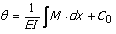where C0 = constant of integration evaluated from the boundary conditions.

The shortening/prolongation due to a direct axial tensile/compressive load is computed fromwhere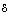= total deformation of the member carrying the axial load
L = original total length of the member
E = modulus of elasticity of the material
A = cross-sectional area of the member

The following formula computes the relative elongation due to a direct axial tensile (compressive) loadWhen a shaft is subjected to a torque, it undergoes a twisting in which one cross-section is rotated relative to the other cross section in the shaft.

The relative twisting angle is defined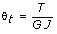The angle of twist is computed fromwhere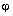= angle of twist (radians)
L = length of the shaft over which the angle of twist is being computed
G = modulus of elasticity of the shaft material in shear

Note:
All applied loads, forces and moments are considered to pass through the center of gravity of the cross-section.

The mass of the beam is not considered. If the consideration of the beam's mass is necessary, then represent it with a distributed load.

Type of beam support

There are two very common beam arrangements. A beam that is supported at one fixed end is commonly referred to as a cantilever. The other is simply supported at two points along the length of the beam. Both of these arrangements are statically defined and are referred to as “determinate.”
The beam that is supported at one end can experience shearing force, longitudinal reaction, twisting, and bending moments.
The beam supported at two points can result in shear forces and reactions due to axial and shear forces.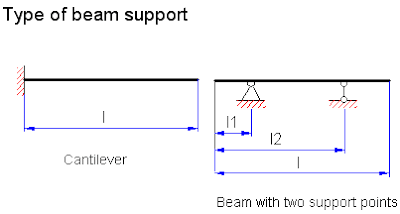Distance to considered cross-section

Distance to the desired cross-section, where the calculation of the force factors and deformations is needed, is defined with “Distance to considered cross-section” variable.Type of cross-sectional shape

For standard structural shapes, click on the table icon and a menu of standard shapes will appear and the software will automatically input the geometric values into the input page. According to the selected cross-section type the following geometrical data must be entered:

Circle:
D diameter mm

Rectangle:
b width mm
h height mm

Shapes:
w depth mm
b flange width mm
t web thickness mm
t1 flange thickness mm
A area mm^2
IX-X moment of inertia mm^4
IY-Y moment of inertia mm^4
SX-X section modulus mm^3
SY-Y section modulus mm^3

Hollow round tube:
d inner diameter mm
D outer diameter mm

Modulus of elasticity in tension

For the part of the stress-strain diagram that is straight, stress is proportional to strain, and the value of E is the constant of proportionality. That is,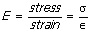This is the slope of the straight-line portion of the diagram. The modulus of elasticity indicates the stiffness of the material, or the resistance to deformation.

Modulus of elasticity in shear

The modulus of elasticity in shear (G) is the ratio of shearing stress to shearing strain. This property indicates a material’s stiffness under shear loading, that is, the resistance to shear deformation. Generally this material property is available from published data. It is commonly provided in this software by clicking on the table icon and selecting the appropriate material.

There is a simple relationship between E, G and Poisson’s ratio:where
E = modulus of elasticity in tension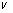= Poisson’s ratio

This equation is valid within the elastic range of the material.

The shearing forces input list “Forces and distributed loads” has the following parameters: distance from the beam’s beginning to the application point “Distance”; length of a distributed load “Length”, (should be 0 for a concentrated force); force/load value; angle of projection’s inclination of the force’s action line on the OYZ plane to Z-axis “a ”. The “Value” sign defines the direction of the force’s action. Force projections on coordinate axis are found using the method of superposition.
Note: Distributed load has unit “lb/in” or "N/mm".

Bending moments

The list of bending moments has the following parameters: distance from the beam’s beginning to the application point “Distance”; value of the moment “Value” and angle of inclination of the moment action plane to Z-axis. The “Value” sign defines the direction of the moment’s action. Moment projections on OXY and OXZ planes are found using the method of superposition.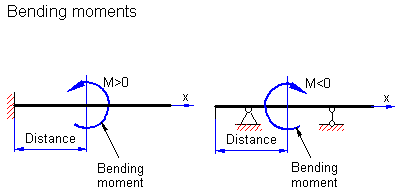Twisting moments

“Twisting moments” list is used for twisting moments input. The list has the following parameters:
distance from the beam’s beginning to the application point, “Distance”, in,
value of the moment, “Value”, lb.in. or N.mm The sign defines the direction of application. The default is shown in the corresponding graphic help.